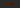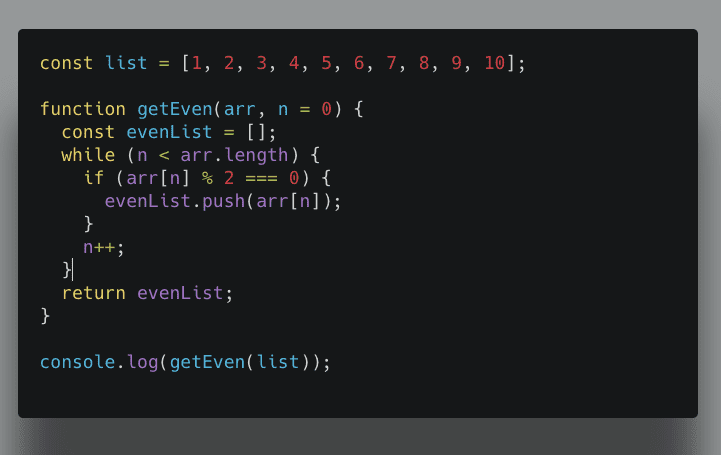# How to use Modulo (%) operator in JavaScript### Modulo operator helps us to find the remainder of the two numbers.

suppose we have two numbers 5 and 2 where `number 1 is dividend` and `number2 is a divisor` ( `number 1 % number 2 = Remainder`).

`````` // 5 mod 2 = 1

5 % 2 = 1 // 1 is remainder

// 10 mod 2 = 0

10 % 2 = 0 //0 is remainder

//9 mod 3  = 0

9 % 3 = 0 // 0 is remainder``````

By using the modulus we can easily find the even numbers and odd numbers in the list.

### How to find even numbers using the modulo operator?Happy coding…

## Top Udemy Courses##### JavaScript - The Complete Guide 2020 (Beginner + Advanced)
45,614 students enrolled
52 hours of video content
View Course##### React - The Complete Guide (incl Hooks, React Router, Redux)
284,472 students enrolled
40 hours of video content
View Course##### Vue JS 2 - The Complete Guide (incl. Vue Router & Vuex)
130,921 students enrolled
21 hours of video content
View Course